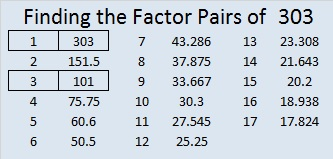# 303 and Level 1

• 303 is a composite number.
• Prime factorization: 303 = 3 x 101
• The exponents in the prime factorization are 1 and 1. Adding one to each and multiplying we get (1 + 1)(1 + 1) = 2 x 2 = 4. Therefore 303 has exactly 4 factors.
• Factors of 303: 1, 3, 101, 303
• Factor pairs: 303 = 1 x 303 or 3 x 101
• 303 has no square factors that allow its square root to be simplified. √303 ≈ 17.407Print the puzzles or type the factors on this excel file: 10 Factors 2014-11-24## One thought on “303 and Level 1”

1.Paula Beardell Krieg

I’ve gotten a bit addicted to checking on number pairs before bedtime. This is a good one.

This site uses Akismet to reduce spam. Learn how your comment data is processed.